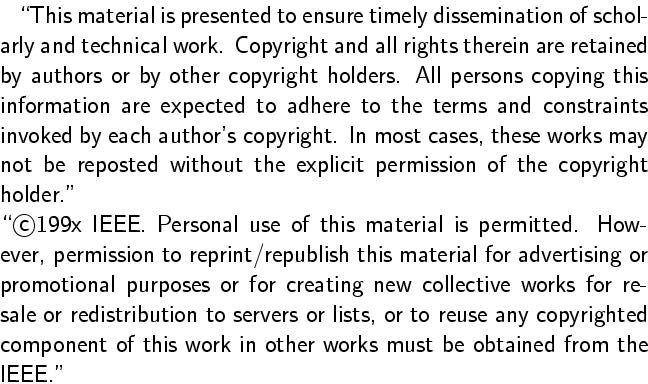Gunther Reißig, Holger Boche, and Paul I. Barton.
On inconsistent initial conditions for linear time-invariant differential-algebraic equations.
IEEE Trans. CAS, Part I, vol. 49, no. 11, Nov. 2002, pp. 1646-1648.
Full text. (Definitive publication; restricted access.)
Full text. (Free access.)Abstract:
Given an arbitrary initial value x0- for the differential-algebraic equation A x'(t)+B x(t) = f(t), an initial value x0+ can be selected from among all consistent initial values by means of the Laplace transform. We show that this choice is the only one that fulfills some simple, physically reasonable assumptions on linear systems' behavior. Our derivation is elementary compared to previous justifications of the above Laplace transform based method. We also characterize x0+ by means of a system of linear equations involving A, B, derivatives of f, and x0-, which gives a new method to numerically calculate x0+.
BibTeX entry:
```@article{ReissigBocheBarton02,
AUTHOR = {Rei{\ss}ig, Gunther and Holger Boche and Paul I. Barton},
TITLE = {On inconsistent initial conditions for linear time-invariant differential-algebraic equations},
JOURNAL = {IEEE Trans. Circuits Systems I Fund. Theory Appl.},
VOLUME = {49},
YEAR = {2002},
NUMBER = {11},
PAGES = {1646-1648},
doi = {10.1109/TCSI.2002.804552}
}
```

Impressum und Haftungsausschluß